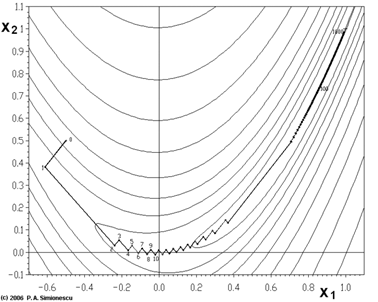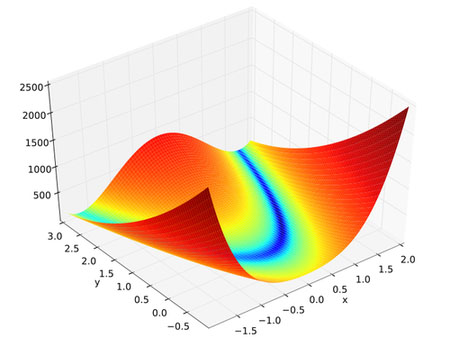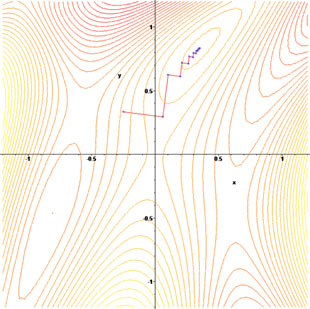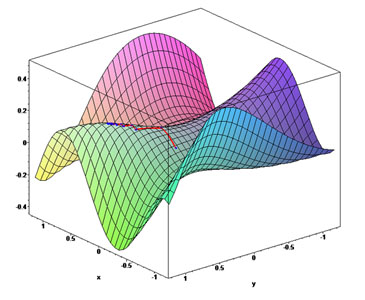『1』名字释义

『2』函数值下降最快的方向是什么

$f(x) = f({x_k}) + \alpha g_k^T{d_k} + o(\alpha )$

$({a_1}{b_1} + {a_2}{b_2} + \cdots + {a_n}{b_n}) \le \sqrt {(a_1^2 + a_2^2 + \cdots + a_n^2)} \sqrt {(b_1^2 + b_2^2 + \cdots + b_n^2)}$

$\frac{{{a_1}}}{{{b_1}}} = \frac{{{a_2}}}{{{b_2}}} = \cdots = \frac{{{a_n}}}{{{b_n}}}$

$\left| {d_k^T{g_k}} \right| \le \left\| {{d_k}} \right\|\left\| {{g_k}} \right\|$

『3』缺点

『4』感受一下它是如何“慢”的$f({x_1},{x_2}) = {(1 - {x_1})^2} + 100 \cdot {({x_2} - {x_1}^2)^2}$『5』为什么“慢”

$\nabla f{({x_k} + {\alpha _k}{d_k})^T}{d_k} = 0$ ，即 $g_{k + 1}^T{d_k} = 0$

$g_{k + 1}^T{d_k} = g_{k + 1}^T( - {g_k}) = - g_{k + 1}^T{g_k} = - d_{k + 1}^T{d_k} = 0 \Rightarrow$ $d_{k + 1}^T{d_k} = 0$

$\cos \theta = \frac{{{d_k}^T{d_k}}}{{\left\| {{d_k}} \right\|\left\| {{d_k}} \right\|}} = \frac{0}{{\left\| {{d_k}} \right\|\left\| {{d_k}} \right\|}} = 0\;$
=> $\theta = \frac{\pi }{2}$

『6』优点

『7』收敛性及收敛速度

• 引用
（1）https://en.wikipedia.org/wiki/Cauchy%E2%80%93Schwarz_inequality
（2）https://en.wikipedia.org/wiki/Gradient_descent
（3）https://en.wikipedia.org/wiki/Rosenbrock_function
（4）https://en.wikipedia.org/wiki/Gradient_descent
[原创] 再谈 梯度下降法/最速下降法/Gradient descent/Steepest Descent

### 3 thoughts on “[原创] 再谈 梯度下降法/最速下降法/Gradient descent/Steepest Descent”

• 2016 年 10 月 12 日 at 03:08
Permalink

选修了optimization的课程，由于本来这方面的知识就比较晦涩加之英文难以理解。基本将博主的几片最优化的文章看了一遍，语言通俗，让我理解了许多上课看书无法理解的东西

Reply
• 2014 年 12 月 25 日 at 20:18
Permalink

博主写的很好，很清晰。

Reply
• 2016 年 09 月 18 日 at 16:04
Permalink

谢谢楼主，写得很详细，帮助了我这种初学者。

Reply